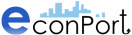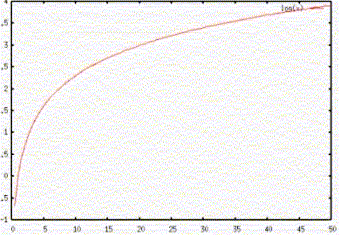http://www.econport.org   A Digital Library for Microeconomics Education Copyright © 2006 Experimental Economics Center, Georgia State University. All rights reserved.Decision-Making Under Uncertainty - Basic Concepts

On the previous page, you were asked how much you would be willing to pay to participate in the following gamble:

Suppose you were made an offer. A fair coin would be tossed continuously until it turned up tails. If the coin came up tails on the nth toss, you would receive \$2n, i.e. if it came up tails on the 5th toss, you would receive \$25 = \$32.

Of course, the first step is to calculate the expected value of the gamble:

The probability of the coin turning up tails on the nth toss (which is, of course, equal to the probability of it turning up heads) equals 1/2n. So the expected value of the gamble would be:
(1/2) * 2 + (1/4) * 4 + (1/8) * 8 + (1/16) * 16 + .....
= 1 + 1 + 1 + 1 + ...... and on till infinity.

The expected value of this gamble is infinity. Certainly a lot more than anyone would be willing to pay for it; in fact, it would be hard to find anyone willing to pay even \$100 to participate in this gamble with an infinite expected value.

This puzzle,which has now become famous as the St. Petersburg Paradox, deeply troubled Swiss mathematician Nicholas Bernoulli, so in 1738 he asked his cousin, Daniel Bernoulli, an even more illustrious mathematician, how it could be explained.

Note: If you are curious about the St. Petersburg paradox in real life, you can see the section on an experimental discussion of the St. Petersburg Paradox.

Bernoulli's Solution To The St. Petersburg Paradox

Daniel Bernoulli's solution lay in the realization that people's "utility", or the subjective, internal value they attach to an additional unit of money is determined by how much money they already have - the value a homeless person would attach to a hundred-dollar bill is far more than Bill Gates would.

Bernoulli hypothesized that a person's utility from money was a logarithmic function of the amount of money, of the form:

u(w) = k log(w) + constant

where w represents the amount of money, or wealth, and k is a parameter.

Why a logarithmic function? Because its value increases at a decreasing rate as the value of its argument increases - in mathematical terms, it is concave.According to Bernoulli, people maximize their expected utility from a gamble, rather than the expected value of the gamble - the sum of the utilities of each payoff, multiplied by their respective probabilities.

E.g.: A table of expected utilities of the payoffs in this case, for a sample function with k = 1 and constant = 0, so u(w) = log(w), would resemble:

 n Probability of Tails on Toss n Payoff Utility of Payoff Expected Utility 1 1/2 \$2 0.301 0.1505 2 1/4 \$4 0.602 0.1505 3 1/8 \$8 0.903 0.1129 4 1/16 \$16 1.204 0.0753 5 1/32 \$32 1.505 0.0470 6 1/64 \$64 1.806 0.0282 7 1/128 \$128 2.107 0.0165 8 1/256 \$256 2.408 0.0094 9 1/512 \$512 2.709 0.0053 10 1/1024 \$1024 3.010 0.0029

The sum of expected utilities, in this case, is not infinite - it converges to about 0.60206. Taking the antilog of this, i.e. 100.60206, we find that it is the utility received from approximately \$4. So a person whose utility function had this simple form would be willing to pay up to \$4 to participate in the gamble.

But Bernoulli's formulation is still not perfect - the paradox becomes a paradox once again if we raise the payoffs to, say, \$102n. Finally, in 1944, mathematicians John Von Neumann and Oskar Morganstern derived a theory of expected utility that resolved many of the peculiarities related to people's behavior under uncertainty.

Continue

References

 Page source: http://www.econport.org/econport/request?page=man_ru_basics2